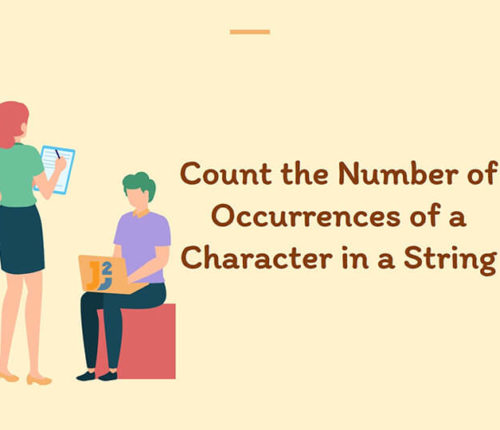# Return second last digit of given number in java

In this topic, we will learn to get the second last digit of a number in Java with the help of examples.
There can be several ways to get second last digit; here we are discussing some solutions.

## Using modulo operator

Here, we are using modulo and divide operators to find the second last digit. The modulo operator gives remainder of a number while divide operator gives quotient as a result.

Output

2

## Using String’s chartAt() method

There is another example in which we can convert the number into a string and then using `charAt()` method we get second last value after that result convert back to number using `Character` class.

Output

2

That’s all about how to return second last digit of given number in java.

import_contacts

### You may also like:## Related Posts

•05 April

### Count occurrences of Character in String in Java

Table of Contents1. Using String Library Methods2. Using Recursion3. Using Hashing ConceptUsing ArraysUsing Collections (Map) 4. Using Java 8 Features In this article, we will look at a problem: Given an Input String and a Character, we have to Count Occurrences Of character in String in java. For Example, If the Given String is : […]

•24 December

### How to capitalize first letter in java

Learn about how to capitalize first letter in java.

•09 October

### Java program to find first and last digit of a number

Table of ContentsAlgorithmUsing while loopUsing log() and pow() methodsUsing while loop and pow() method In this article, we are going to find first and last digit of a number in Java. To find first and last digit of any number, we can have several ways like using modulo operator or pow() and log() methods of […]

•03 October

### Happy Number program in Java

Table of ContentsWhat is a Happy Number?Using HashsetAlgorithmExampleUsing slow and fast pointersAlgorithmExample In this article, we are going to learn to find Happy Number using Java. Let’s first understand, what is Happy Number? What is a Happy Number? A number which leaves 1 as a result after a sequence of steps and in each step […]

•30 September

### Find Perfect Number in Java

Table of ContentsIterative approachRecursive approach In this article, we are going to find whether a number is perfect or not using Java. A number is called a perfect number if the sum of its divisors is equal to the number. The sum of divisors excludes the number. There may be several approaches to find the […]

•28 September

### How to find Magic Number in Java

Table of ContentsWhat is a Magic Number?Algorithm for Magic NumberExample to find Magic NumberAnother Example To find Magic Number In this article, we are going to learn to find Magic Number using Java. Let’s first understand, what is Magic Number? What is a Magic Number? A number which leaves 1 as a result after a […]

## Subscribe to our newletter

Get quality tutorials to your inbox. Subscribe now.